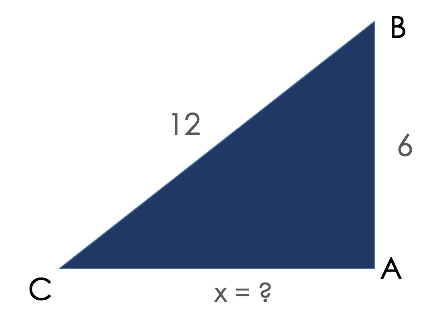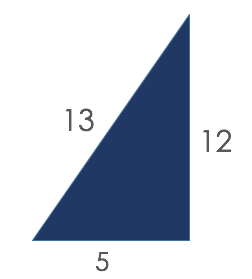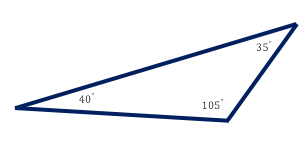# Triangles

In this lesson, we will look at triangle constructions as we focus on two very important theorems regarding sides and angles.

Let us begin with the Triangle Inequality Theorem which states that:

the sum of the lengths of any two sides of a triangle
is greater than the length of the third side.### Example

We can use this theorem to determine if the following lengths are legs of triangles.

4, 9, 5 and 9, 5, 5

*Strategy – Choose the smallest two of the three sides and add them together. Then, compare that sum to the third side.

4, 9, 5

4 + 5 > 9 (THIS IS FALSE)

Since the sum is not greater than the third side, this is NOT a triangle.

9, 5, 5

5 + 5 > 9 (THIS IS TRUE)

Sine the sum IS greater than the third side, this is a triangle.

### Example

A triangle has side lengths of 6 and 12; what are the possible lengths of the third side?6 + 12 = 18 (for the side to make the inequality theorem work, 6 + 12 > x)

12 – 6 = 6 (for the side to make the inequality theorem work, 6 + x > 12)

This means that the third side must be between 6 and 18. (Difference and sum of the given sides)

Solution: 6 < x < 18

Here are two more Triangle Theorems involving side lengths of triangles.

1. If one side of a triangle is longer than another side,
then the angle opposite the longer side is the larger angle.2. If one angle of a triangle is larger than another angle,
then the side opposite the larger angle is the longer side.Watch this video to see examples of what is behind the Triangle Inequality Theorem.The triangle inequality theorem deals with the side lengths. Another theorem, called the Triangle Angle Sum Theorem states that:

the sum of the angles in any triangle is 180°.
No triangles can have two obtuse angles.

Is it possible to construct a triangle with angles measuring 61°, 33°, and 86°?

Use the Triangle Angle Sum Theorem to test these angle measures. Add the angle measures: 61 + 33 + 86 = 180. A triangle with these measures is possible.

## Practice

#### Are these a triangle or not?

1. Triangle with side lengths 3, 2, and 1
2. Triangle with side lengths 10, 12, 14
3. Triangle with angles 20, 70, and 90
4. Triangle with angles 60, 60, and 70

(source)